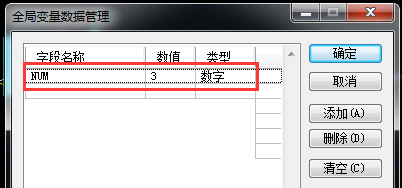﻿ 金字塔日内限制交易次数略源码-金字塔模型 －程序化交易（CXH99.COM）

# 金字塔日内限制交易次数略源码[金字塔模型]

多种全局变量的区别介绍：http://www.weistock.com/WeisoftHelp/index.html?zbgs003.htm

一、日内限制交易次数范例（图表程序化）

//该策略适用于图表程序化交易
//该策略适用于分钟周期
//此范例仅供显示，请勿直接用于入市交易

VARIABLE：NUM=0;                            // 全局变量,来控制当天交易次数
CS:=3;                                              //限定一天最多交易3次

MA5:=MA(CLOSE, 5);
MA20:=MA(CLOSE, 20);

COND1:=CROSS(MA5,MA20);
COND2:=CROSS(MA20,MA5);

IF COND2 AND HOLDING>0 THEN SELL(1,1,MARKET);     //平仓

IF COND1 AND HOLDING=0 AND NUM<CS THEN             //开仓
BEGIN
NUM:=NUM+1;
END

IF TIME=CLOSETIME(0) THEN NUM:=0;             // CLOSETIME(0)是取商品期货最后一节的交易时间,收盘的同时,NUM赋值为0。

二、日内限制交易次数范例（后台程序化）

点击工具--数据管理--全局变量，创建一个全局变量NUM，初始值设为3，表示交易次数限定为3次。此主题相关图片如下：11.png//该策略适用于图表程序化交易
//该策略适用于分钟周期
//使用单值全局变量，来限定一天只交易3次
CS:=3;//限定一天最多交易3次
MA5:MA(CLOSE, 5);
MA20:MA(CLOSE, 20);
COND1:=CROSS(MA5,MA20);
COND2:=CROSS(MA20,MA5);

IF COND2 AND THOLDING>0 THEN TSELL(1,1,LMT,CLOSE);       //平仓

NUM:=EXTGBDATA(\'NUM\');                                                      //获取单值全局变量,来控制当天交易次数
IF COND1 AND THOLDING=0 AND NUM< CS  THEN                    //开仓

BEGIN
EXTGBDATASET(\'NUM\',NUM+1);
END

IF TIME=CLOSETIME(0) THEN EXTGBDATASET(\'NUM\',0); // CLOSETIME(0)是取商品期货最后一节的交易时间,收盘时,NUM赋值为

{别忘了将本网告诉您身边的朋友，向朋友传达有用资料，也是一种人情，你朋友会感谢你的。}

(注：由于人数限制，QQ或微信请选择方便的一个联系我们就行，加好友时请简单备注下您的需求，否则无法通过。谢谢您！)

【字体： 】【打印文章】【查看评论

没有相关内容